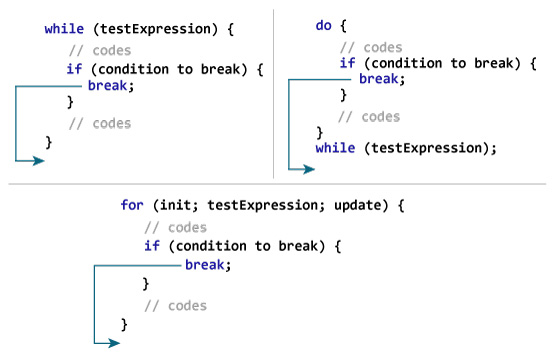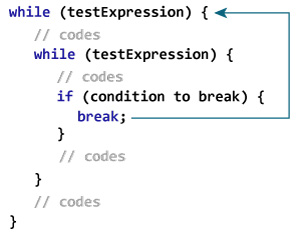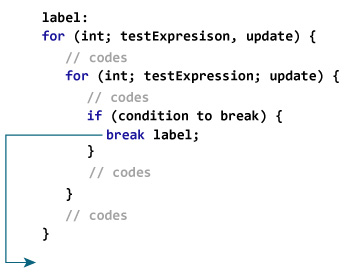# blog posts# Suppose you are working with circles. Sometimes you need to skip some commands inside the loop or end the loop immediately without checking the condition.

In such cases, break and continue commands are used. The break statement terminates the loop immediately and program control goes after the loop. This is often used with decision statements (if… else).

The break structure is:

break;

## How does the break command work?Example 1: break in Java

1. class Test {
2. public static void main (String [] args) {
3. for (int i = 1; i <= 10; ++ i) {
4. if (i == 5) {
5. break;
6. }
7. System.out.println (i);
8. }
9. }
10. }

Output

1

۲

3

4

In the above program, when the value of i equals 5, we put the expression i == 5 in parentheses if. Then, the break command is executed and terminates the for loop.

Example 2: The phrase break in Java

The following program calculates the sum of the numbers entered by the user until he enters a negative number.

The Scanner object is used to get input from the user.

1. import java.util.Scanner;
2. class UserInputSum {
3. public static void main (String [] args) {
4. Double number, sum = 0.0;
5. Scanner input = new Scanner (System.in);
6. while (true) {
7. System.out.print (“Enter a number:”);
8. number = input.nextDouble ();
9. if (number <0.0) {
10. break;
11. }
12. sum + = number;
13. }
14. System.out.println (“Sum =” + sum);
15. }
16. }

Output

Enter a number: 3.2

Enter a number: 5

Enter a number: 2.3

Enter a number: 0

Enter a number: -4.5

Sum = 10.5

In the above program, the while loop condition is always true and runs until the user enters a negative number. If the user enters a negative number, the command is executed inside the if body, which terminates the while loop.

If there are nested loops, the break terminates the innermost loop.Here, the term break terminates the inner while loop and the program control jumps to the outer loop.

## Labeled break command

The break statement we have discussed so far is the unmarked form break, which terminates the innermost loops for, while, do… while, and switch. There is another form of break command that has a break tag that can be used to terminate the outer loop.

### How does the labeled break command work?Here, the label is an identifier. When you run break, the labeled command ends, and the program control jumps to after the labeled command.

Another example:

1. while (testExpression) {
2. // codes
3. second:
4. while (testExpression) {
5. // codes
6. while (testExpression) {
7. // codes
8. break second;
9. }
10. }
11. // control jumps here
12. }

When running break, the program control jumps to the phrase labeled second.

Example 3: Labeled break in Java structure

1. class LabeledBreak {
2. public static void main (String [] args) {
3. first:
4. for (int i = 1; i <5; i ++) {
5. second:
6. for (int j = 1; j <3; j ++) {
7. System.out.println (“i =” + i + “; j =” + j);
8. if (i == 2)
9. break first;
10. }
11. }
12. }
13. }

Output

i = 1; j = 1

i = 1; j = 2

i = 2; j = 1

#### Here is another method of the above program. break In the following program, terminates the second tagged phrase and the program control jumps to the second tagged phrase.

1. class LabeledBreak {
2. public static void main (String [] args) {
3. first:
4. for (int i = 1; i <5; i ++) {
5. second:
6. for (int j = 1; j <3; j ++) {
7. System.out.println (“i =” + i + “; j =” + j);
8. if (i == 2)
9. break second;
10. }
11. }
12. }
13. }

Output

i = 1; j = 1

i = 1; j = 2

i = 2; j = 1

i = 3; j = 1

i = 3; j = 2

i = 4; j = 1

i = 4; j = 2

The break command is also used to terminate the switch.